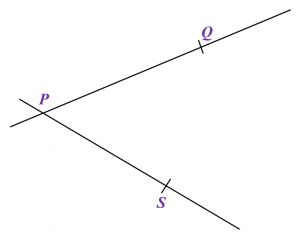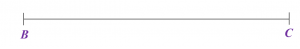7.2.2 Geometrical Constructions, PT3 Focus Practice

7.2.2 Geometrical Constructions, PT3 Focus Practice 2

Question 4:
Diagram below shows a parallelogram PQRS. The point T lies on PQ such that ST is perpendicular to PQ.Using only a ruler and a pair of compasses, construct parallelogram PQRS, beginning from the lines PQ and PS provided in the answer space.

:Solution
:Question 5:
Diagram below shows a triangle ABC.(a) Using only a ruler and a pair of compasses, construct triangle ABC, beginning with the straight line BC in the answer space.
(b)   Hence, measure the length, in cm, of BA.